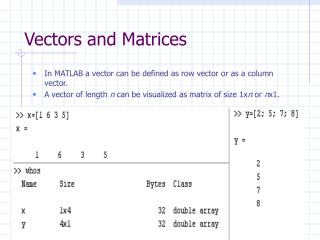DownloadDownload PresentationVectors and Matrices

# Vectors and Matrices

Download Presentation## Vectors and Matrices

- - - - - - - - - - - - - - - - - - - - - - - - - - - E N D - - - - - - - - - - - - - - - - - - - - - - - - - - -
##### Presentation Transcript

1. Vectors and Matrices • In MATLAB a vector can be defined as row vector or as a column vector. • A vector of length n can be visualized as matrix of size 1xn or nx1.

2. Vectors and Matrices • Matrices can be of different sizes, either square matrices of size NxN or rectangular NxM. • Note that vectors are special case of matrices.

3. Some Special Vectors and Matrices

4. Matrix Calculations • Addition • Multiplication • Inverse

5. Solving Simultaneous EquationsAx=b • A=matrix of coefficients, • x = vector of variables, • b = vector of values. • In matrix form the above system and its solution can be written as • In MATLAB we write as x = inv(A)*b

6. Loops • Two different types of loops exist in MATLAB. • for and • while • Their structure is as shown here

7. for loop • A simple for loop to calculate and display squares of numbers from 1 to 5.

8. for loop (contd.) • We can write the same for loop with some change. Now we are saving the results in a vector named ‘result’. Note that the squared values will be saved in the ‘result’ vector. The vector must be initialized before assigning any value to it. Note that this is the case for vectors and matrices only. We don’t need to initialize scalars in MATLAB.

9. for loop (contd.) • The control index of the for loop shown in the previous slides was incremented by ‘1’. We can increment the control index by any arbitrary value as required. • In the following example odd numbers from 1 to 9 are printed.

10. while loop • While loop continue to execute if the given condition is true. • A condition is true if it is not equal to ‘0’. • A simple while loop to display numbers from 1 to 10 is shown here. In this while loop at each iteration the value of ‘i’ is incremented after the value is printed.

11. Logical Operators • AND, OR, and INVERT logical operations can be performed in MATLAB. • The symbols used for these operators are ‘&&’ , ‘||’, and ‘~’ respectively for AND, OR, and INVERT. • A logical operation always results in a ‘1’ or ‘0’.

12. Some more logical operators • Other logical operators in MATLAB include • Note that the results of these operations are also either ‘1’ or ‘0’.

13. Examples: logical operations • Lets say, a=1, b=2, and c=1. Then, • A<b results in TRUE I.e. ‘1’ • A>b results in FALSE I.e. ‘0’ • A==b results in FALSE I.e. ‘0’ • A==c results in TRUE I.e. ‘1’ • A==c && a<=b results in ‘1’. • Several examples can be used to show the usage of these operators.

14. while loop revisited • Now we can show the usage of these logical operations in the while loop as condition. • Say a=1, then the following code results in the execution of loop until the condition a<b gets FALSE.

15. if statement • if statement checks whether the condition given to it is TRUE or FALSE. • If the condition evaluates to TRUE the <statements> are executed otherwise not. • Unlike the for and while loops the <statements> inside the if statement are executed only once.

16. if-else statement • If-else is used if we want to perform two different tasks depending upon the condition is TRUE or FALSE.

17. We wish to use “if” and “else” statement to decide upon if the input integer is an odd or an even number. The Matlab code for the purpose is shown below:

18. Functions in Matlab • Functions are actually computer routines(programs) to perform specific task. • Matlab is rich in built-in function • A function is called, user gives specific input(s) to the function, which generates the required output(s).

19. Example of built-in functions • Our objective is to calculate mean or average of certain vector ‘x’. • We call the built in function “mean” of Matlab and give ‘x’ as an input to the function. • The mean is calculated and is stored in variable ‘y’.

20. We can also built our own functions and call them when required. • The functions are the m-files. • For example for a given quadratic equation we wish to calculate the roots of equation. So we build our own function “call” and use the quadratic formula to solve for x1 and x2.

21. In our main program the function is called and the inputs ‘a’, ‘b’ and ‘c’ are given to the function which calculates the required roots

22. Plotting in Matlab • Matlab uses “plot” command to plot the curves with different line style and colors • Example follows:

23. Thank You